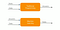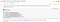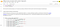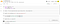# Learning Machine Learning — How to Code without Learning Coding

The title looks like a self-contradictory statement. If a machine can learn, why we have to learn machine learning? If we want to writing coding, how come can we code without learning coding?

# The 5th generation of artificial intelligence

Why we still have to learn machine learning? It is because so far artificial learning is still at its 4th generation (Yiu, 2019). We have started from the 1st generation which focused on automation to the 4th generation which emphasizes machine learning. These two approaches are exactly the opposite. Automation requires a hard-wired instruction (rules) provided by the programmer. Machine Learning, on the contrary, tries to identify the rules from experience (training) (Figure 1). For example, last week we have tried to train the computer to differentiate two people by analyzing their photos (Yiu, 2021). However, we still have to take the initiative to train the machine to learn something, so that it can perform the task. The 5th generation of artificial intelligence is a self-learning machine. It is more like a human baby who can learn by himself or herself even their parents do not train. Robots are now trying to learn by itself. When the 5th generation of AI can be successful, we do not have to learn machine learning anymore.Figure 1 Difference between traditional programming and machine learning. Source: https://medium.com/@ndang.nguyen94/machine-learning-3e833be54bdf

Code without Learning Coding

In the current 4th generation of artificial intelligence, we have 4 levels of involvement. The upper level is to train the machine by experiential learning. In our previous article, when we provide a lot of photos to the computer to learn how to differentiate two persons, we are training it by providing experience. It does not require any coding, as programmers from the Teachable Machine have prepared a user-friendly apps for us to train.

The second level of our involvement is to combine or adapt existing libraries of coding to fit our purposes. If the interface is well written, theoretically users can code without learning how to code. If you are interested in this level of involvement, this article is going to provide some introductory information for exploration in the next section.

Then the third and fourth levels are to learn coding and/or machine code to directly instruct the machine with the learning algorithm. But then it requires learning programming languages, such as Python, and statistical concepts, such as linear regression and logit regression. In fact, Python can also be considered as a language using a lot of libraries of machine codes to instruct the machine to run.

# Google Colaboratory

Google has provided a user-friendly interface called Colab for us to run the libraries of coding for machine learning. Provided that there can have enough libraries for us to combine and adapt easily, then we can code without learning coding.

For example, in our previous exercise, we have used the apps to train the computer to recognize people. If you want to know how the coding can carry out the process, here is a simplified version of a combination of several libraries of coding that can train a machine to differentiate a horse and a human by analyzing photos at Google Colab platform (Colab, 2020: https://youtu.be/0kYIZE8Gl90).

Once you login the Colab platform (Figure 2), it provides an interface for running Python codes on Google Cloud and writing text in-between the codes. If you click open this libraries of Lab5 Using Convolutions With Complex Images, the first library is the python codes to use Operating System (OS) libraries, giving you access to the file system, and the zipfile library allowing you to unzip the data. The text explains what the codes are, but we do not have to understand what they are, simply press the black circle at the left hand side of the block, it runs the libraries to download and extract the horse-and-human photos zip file from the base directory `/tmp/horse-or-human`Figure 2 Google Colab Lab5 Using Convolutions with Complex Images. Source: https://colab.research.google.com/github/lmoroney/mlday-tokyo/blob/master/Lab5-Using-Convolutions-With-Complex-Images.ipynb#scrollTo=o-qUPyfO7Qr8

Each coding block can be considered a library of Python performing different functions. For example, The top block in Figure 3 shows how to import tensorflow as tf, which is a google platform for machine learning coding. Then the second block shows how to use tf.keras to train the computer to classify photos. Keras is a deep learning API written in Python, running on top of the machine learning platform TensorFlow. As we are not going to talk about coding in this article, so you can run the libraries by clicking the [ ] on the top left corner of each block.Figure 3 Another two libraries of Python coding.

# An Introduction to Colab libraries

If you want to know how to adapt the libraries to fit your purposes, then you may have to learn Colab from the very beginning. Here is the simplest introduction to Colab coding — running a simple regression to predict Y from a linear polynomial of X.

For example, if we provide several pairs of X and Y to train the computer to predict Y by giving X, then it can be done by a single layer Artificial Neural Network (which is equivalent to a simple regression). Let’s go into details to see how to do that at Colab. [A Youtube is also prepared https://youtu.be/sCGuMx_5QNs]

Example 1: Simple Regression: Predict Y from X

The following link (Say hello to the “Hello, World” of machine learning) provides the full set of the Python codes for a simple regression and their explanations. It also provides some background information of google Colab.

But if we do not want to know what the codes are doing, then it just requires 6 libraries (blocks) of Python codes to achieve the purpose, as shown in Figure 4.

The 1st block simply import the required tools, such as Tensorflow and Keras.

The 2nd block defines the model as a single layer neural network (i.e. a simple regression).

The 3rd block defines the optimizer and the loss function.

The 4th block provides the data (X-Y pairs).

The 5th block trains the computer and

the 6th block tests it with a new X to predict Y.Figure 4 Python libraries to run a simple regression at Colab.

After running all the 6 libraries, it predicts Y=209 when X=100. Since we know that the polynomial equation is Y=3X+1, the prediction is not good. The reason is because we provide very few data pairs for training, and the training epochs are small.

If you compare the 6 blocks of coding in Figure 4 with that in the google site, you will find that I intentionally change the code a bit to show you how to adapt the coding to our purpose without learning how to code. For example, I change the model name from model to model1, the number of data pairs are fewer, and the number of epochs is also reduced to 50. You may try replace the xs and ys by your own set of data, say Y=0.5X+13, i.e. (1,13.5), (2,14), (3,14.5), (4, 15), …, see how would the result be when X=100.

# Other libraries

If we do not want to learn how to code, then there must have sufficiently large number of existing libraries for us to combine and adapt to achieve our purposes. It may take much longer time for the developers to create more libraries, and provide a more user-friendly interface to adapt the libraries to different purposes. You may help to develop the libraries and the interface. Now there are quite a large number of libraries at Colab and Github, you may also help contribute to build more.

Here are two more examples for your references. One is for recognizing clothes by photos, and the other is for multiple regression of predicting housing prices.

Example 2: Classification: Recognize an object by photos

Example 3: Multiple Regression: Predict house prices by attributes

References

Colab (2020) Machine Learning Foundations: Ep #5 — Classifying real-world images, 21 May. https://youtu.be/0kYIZE8Gl90

Yiu, C.Y. (2019) From Automation to Machine Learning, Medium, Jan 4. https://ecyy.medium.com/from-automation-to-machine-learning-c61fefe483f5

Yiu, C.Y. (2021) Learning Machine Learning — Training People to Train a Machine, Medium, Feb 2. https://ecyy.medium.com/learning-machine-learning-7e273c1a4728

ecyY — easy to understand why, easy to study why. Finding the truths scientifically is the theme.

## More from ecyY

ecyY — easy to understand why, easy to study why. Finding the truths scientifically is the theme.